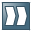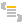Show TOC

###Access to Tables#### Procedure

If the BAPI call is successful, it outputs a table containing all the company codes.

1. First, get the table by accessing the table parameter list (getTableParameterList()).

2. Within this list, access the actual table (getTable()). The class JCO.Table contains all methods that are available for JCO.Structure, together with additional methods for navigation in a table. A table can have any number of rows, or can also have no rows. The diagram below shows navigation with the method setRow(), in which the current row pointer is moved to every row in the table in turn. The method getNumRows() specifies how many rows exist in total. Instead of setRow(), you can also use the method nextRow(), as displayed in the lower section of the diagram.Syntax

Accessing a Table

1. `JCO.Table codes = null;`
2. `codes =`
3. `   function.getTableParameterList ().getTable(“COMPANYCODE_LIST“);`
4. `for (int i = 0; i < codes.getNumRows(); i++)   {`
5. `   codes.setRow(i);`
6. `   System.out.println(codes.getString(“COMP_CODE“)  +  ‚\t‘  +`
7. `                                codes.getString(“COMP_NAME“));`
8. `}`
9. ` JCO.Table codes = null;`
10. `codes =`
11. `   function.getTableParameterList ().getTable(“COMPANYCODE_LIST“);`
12. `for (int i = 0; i < codes.getNumRows(); i++, codes.nextRow() ) {`
13. `   System.out.println(codes.getString(“COMP_CODE“)  +  ‚\t‘  +`
14. `                                codes.getString(“COMP_NAME“));`
15. `}`
End of the code.